### Home > CALC > Chapter 7 > Lesson 7.3.1 > Problem7-108

7-108.
1. Find the antiderivative of the following differential equations. Use implicit integration when necessary. Solve your equations for y. Check your answer by differentiating the result. Homework Help ✎

1.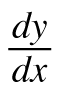= 7x

2.= 7y

3.= ey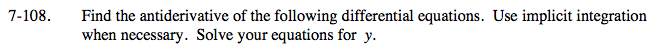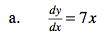y = _______ + C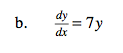Translation: The derivative of y is y times a constant...
What is y?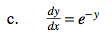Translation: The derivative of y is ey. What is y?

Will absolute value symbols be part of the solution?
Why or why not?

$\frac{dy}{dx}=\frac{1}{e^{y}}$

eydy = dx

$\int e^{y}dy=\int dx\text{ \ \ \ \ Now solve for }y.$